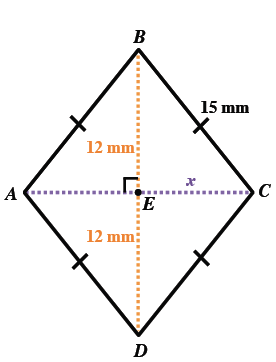### Home > CCG > Chapter Ch7 > Lesson 7.1.4 > Problem7-44

7-44.

In problem 7-41 you learned that the diagonals of a rhombus are perpendicular bisectors. If $ABCD$ is a rhombus with side length $15$ mm and if $BD=24$ mm, then find the length of the other diagonal, $\overline{AC}$. Draw a diagram and show all work.

Make an accurate and well-labeled diagram of the situation.

Use the right triangle $ΔBCE$ and the Pythagorean Theorem to solve for $x$.

$x=18$ mm